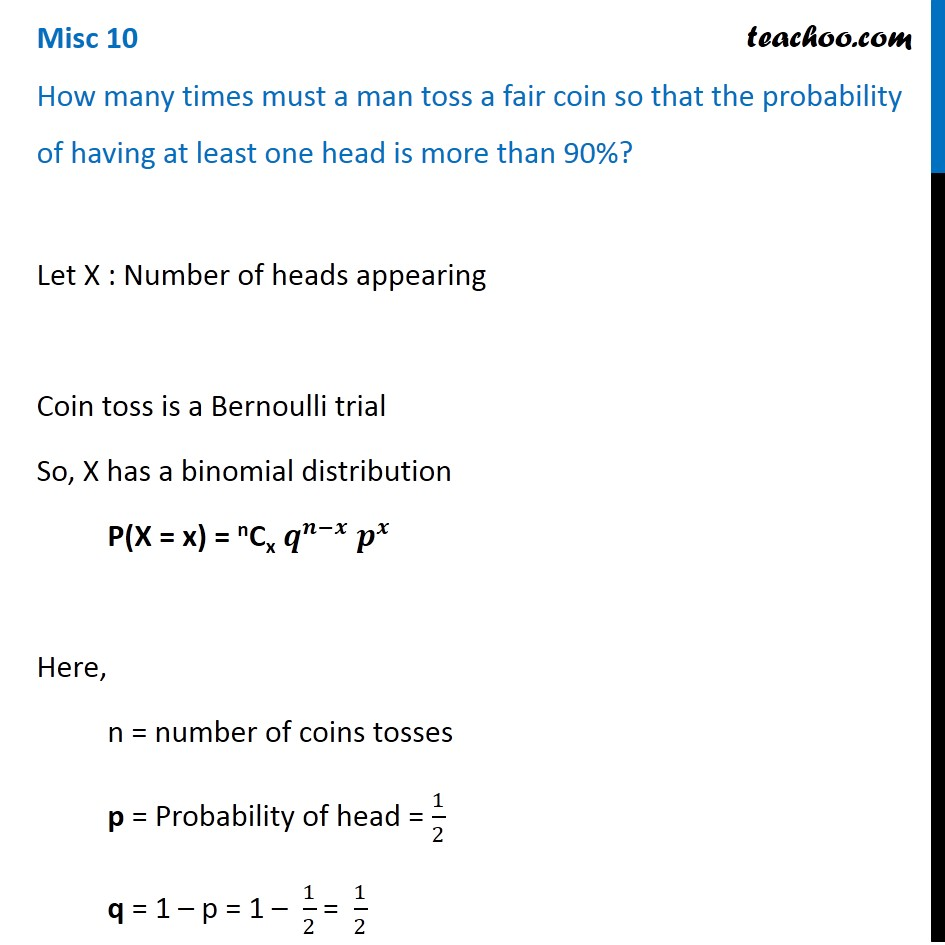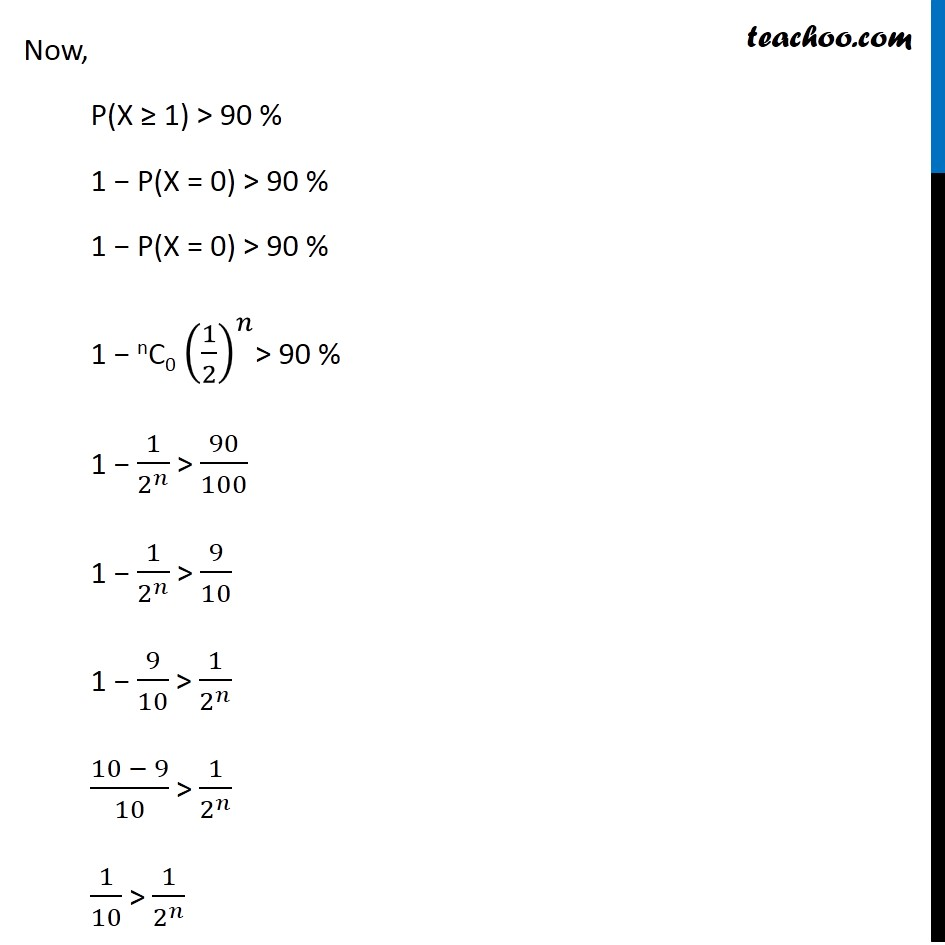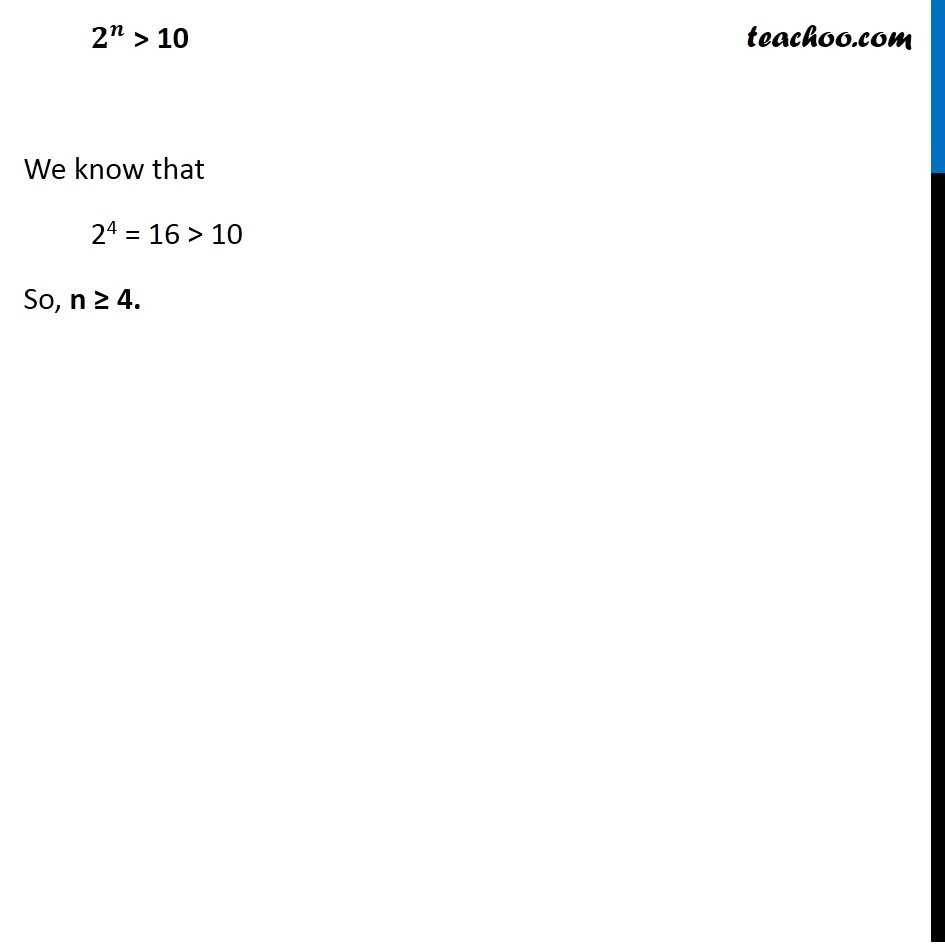Miscellaneous

Chapter 13 Class 12 Probability
Serial order wiseLearn in your speed, with individual attention - Teachoo Maths 1-on-1 Class

### Transcript

Question 5 How many times must a man toss a fair coin so that the probability of having at least one head is more than 90%? Let X : Number of heads appearing Coin toss is a Bernoulli trial So, X has a binomial distribution P(X = x) = nCx 𝒒^(𝒏−𝒙) 𝒑^𝒙 Here, n = number of coins tosses p = Probability of head = 1/2 q = 1 – p = 1 – 1/2 = 1/2 Hence, P(X = x) = nCx (1/2)^𝑥 (1/2)^(𝑛−𝑥) P(X = x) = nCx (1/2)^(𝑛 − 𝑥 + 𝑥) P(X = x) = nCx (𝟏/𝟐)^𝒏 We need to find How many times must a man toss a fair coin so that the probability of having at least one head is more than 90%? So, given P(X ≥ 1) > 90%, we need to find n Now, P(X ≥ 1) > 90 % 1 − P(X = 0) > 90 % 1 − P(X = 0) > 90 % 1 − nC0 (1/2)^𝑛> 90 % 1 − 1/2^𝑛 > 90/100 1 − 1/2^𝑛 > 9/10 1 − 9/10 > 1/2^𝑛 (10 − 9)/10 > 1/2^𝑛 1/10 > 1/2^𝑛 𝟐^𝒏 > 10 We know that 24 = 16 > 10 So, n ≥ 4.